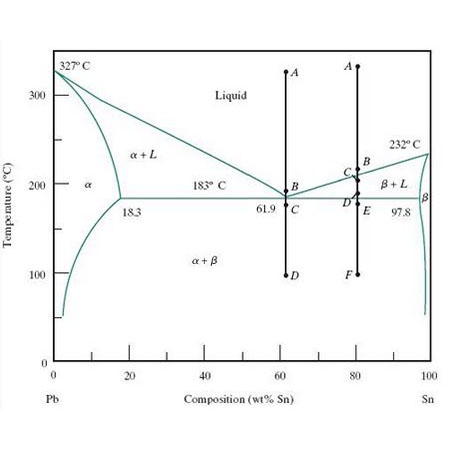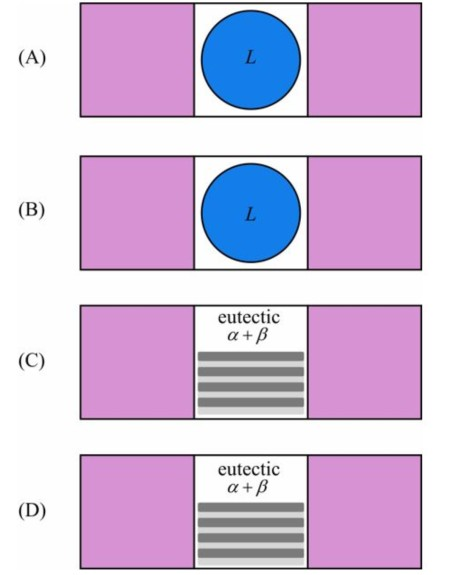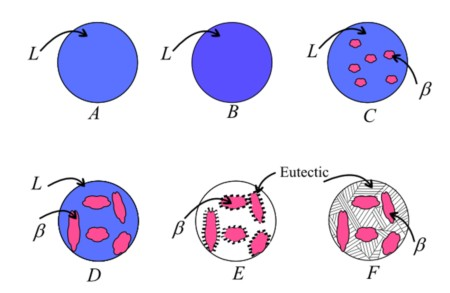# The phase diagram below shows the cooling process for a lead-tin mixture that has 80 wt% tin. For...

## Question:

The phase diagram below shows the cooling process for a lead-tin mixture that has 80 wt% tin. For each point along the cooling line, determine the appropriate microstructure and place it on the figure below. The liquid phase is labeled with an {eq}L {/eq} when it is present in the microstructure. If there are arrows emanating from a phase in a microstructure, use the appropriate solid phase label to identify that phase. Assume that the mixture is cooled slowly.

Consider the cooling of a eutectic mixture of lead and tin, which has 61.9 wt% tin. The cooling line for this mixture is shown below. For each point along the cooling line, determine the appropriate microstructure and place it on the figure below. The liquid phase is labeled with an {eq}L {/eq} when it is present in the microstructure. If there are arrows emanating from a phase in a microstructure, use the appropriate solid phase label to identify that phase. Assume that the mixture is cooled slowly.## Fe-C phase diagram

The iron-carbon phase diagram describes the iron-carbon system of alloys containing up to 6.67% of carbon, discloses the phases compositions and their transformations occurring with the alloys during their cooling or heating.

## Eutectic mixture

A eutectic mixture is a mixture of two or more components which usually do not interact but, at certain ratios, inhibit the crystallization process of one another resulting in a system having a lower melting point than both the components.

## PART B

Point A:

Point A lies in the liquid phase. Therefore the complete microstructure is the liquid state.

Point B:

Point B lies in the liquid phase. Therefore the complete microstructure is the liquid state.

Point C:

At point C, the consumption of the mixture is 61.9% tin. We know that the eutectic mixture of lead and tin alloy contains 61.9% tin. Therefore, the composition is a eutectic composition of {eq}\alpha + \beta {/eq}

Point D:

There is no difference between the microstructure at point C and D because it is an eutectoid mixture and there is no phase change. The microstructure of the eutectic mixture cannot change with temperature change. So, it is also a eutectic composition of {eq}\alpha + \beta {/eq}## PART A